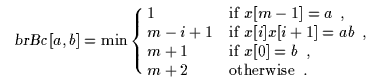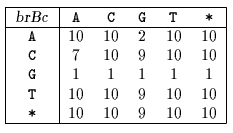Name
• String
• Substring
• Search
Edit

Berry and Ravindran designed an algorithm which performs the shifts by considering the bad-character shift (see chapter Boyer-Moore algorithm) for the two consecutive text characters immediately to the right of the window.

The preprocessing phase of the algorithm consists in computing for each pair of characters (ab) with ab inthe rightmost occurrence of ab in axb. For ab inThe preprocessing phase is in O(m+2) space and time complexity.

After an attempt where the window is positioned on the text factor y[j .. j+m-1] a shift of length brBc[y[j+m],y[j+m+1]] is performed. The text character y[n] is equal to the null character and y[n+1] is set to this null character in order to be able to compute the last shifts of the algorithm.

The searching phase of the Berry-Ravindran algorithm has a O(mn) time complexity.

Main features:

• hybrid of the Quick Search and Zhu and Takaoka algorithms;
• preprocessing phase in O(m+2) space and time complexity;
• searching phase in O(mn) time complexity.

Example:

Preprocessing phaseThe star (*) represents any character in\{A, C, G, T}.
brBc table used by Berry-Ravindran algorithm.

Searching phase

 G C A T C G C A G A G A G T A T A C A G T A C G 1 2 3 4 G C A G A G A G

Shift by: 1 (brBc[G][A])

 G C A T C G C A G A G A G T A T A C A G T A C G 1 G C A G A G A G

Shift by: 2 (brBc[A][G])

 G C A T C G C A G A G A G T A T A C A G T A C G 1 G C A G A G A G

Shift by: 2 (brBc[A][G])

 G C A T C G C A G A G A G T A T A C A G T A C G 1 2 3 4 5 6 7 8 G C A G A G A G

Shift by: 10 (brBc[T][A])

 G C A T C G C A G A G A G T A T A C A G T A C G 1 G C A G A G A G

Shift by: 7 (brBc[G])

 G C A T C G C A G A G A G T A T A C A G T A C G 1 G C A G A G A G

Shift by: 10 (brBc)

The Berry-Ravindran algorithm performs 16 character comparisons on the example.

C